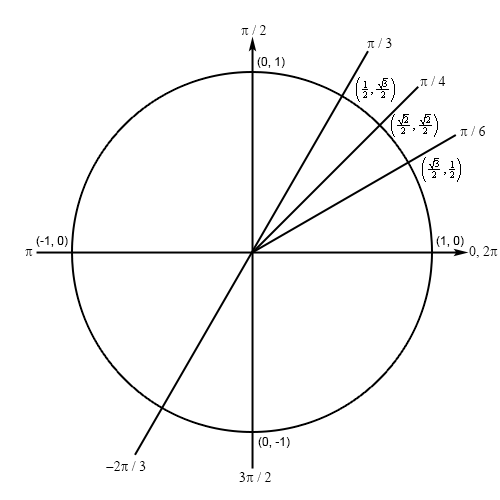Paul's Online Notes
Home / Calculus I / Review / Trig Functions
Show Mobile Notice Show All Notes Hide All Notes
Mobile Notice
You appear to be on a device with a "narrow" screen width (i.e. you are probably on a mobile phone). Due to the nature of the mathematics on this site it is best views in landscape mode. If your device is not in landscape mode many of the equations will run off the side of your device (should be able to scroll to see them) and some of the menu items will be cut off due to the narrow screen width.

### Section 1.3 : Trig Functions

4. Determine the exact value of $$\displaystyle \cos \left( { - \frac{{2\pi }}{3}} \right)$$ without using a calculator.

Show All Steps Hide All Steps

Hint : Sketch a unit circle and relate the angle to one of the standard angles in the first quadrant.
Start Solution

First we can notice that $$- \pi + \frac{\pi }{3} = - \frac{{2\pi }}{3}$$ so (recalling that negative angles rotate clockwise and positive angles rotation counter clockwise) the terminal line for $$- \frac{{2\pi }}{3}$$ will form an angle of $$\frac{\pi }{3}$$ with the negative $$x$$-axis in the third quadrant and we’ll have the following unit circle for this problem.Hint : Given the obvious symmetry in the unit circle relate the coordinates of the line representing $$- \frac{{2\pi }}{3}$$ to the coordinates of the line representing $$\frac{\pi }{3}$$ and use those to answer the question.
Show Step 2

The line representing $$- \frac{{2\pi }}{3}$$ is a mirror image of the line representing $$\frac{\pi }{3}$$ and so the coordinates for $$- \frac{{2\pi }}{3}$$ will be the same as the coordinates for $$\frac{\pi }{3}$$ except that both coordinates will now be negative. So, our new coordinates will then be $$\left( { - \frac{1}{2}, - \frac{{\sqrt 3 }}{2}} \right)$$ and so the answer is,

$\cos \left( { - \frac{{2\pi }}{3}} \right) = - \frac{1}{2}$